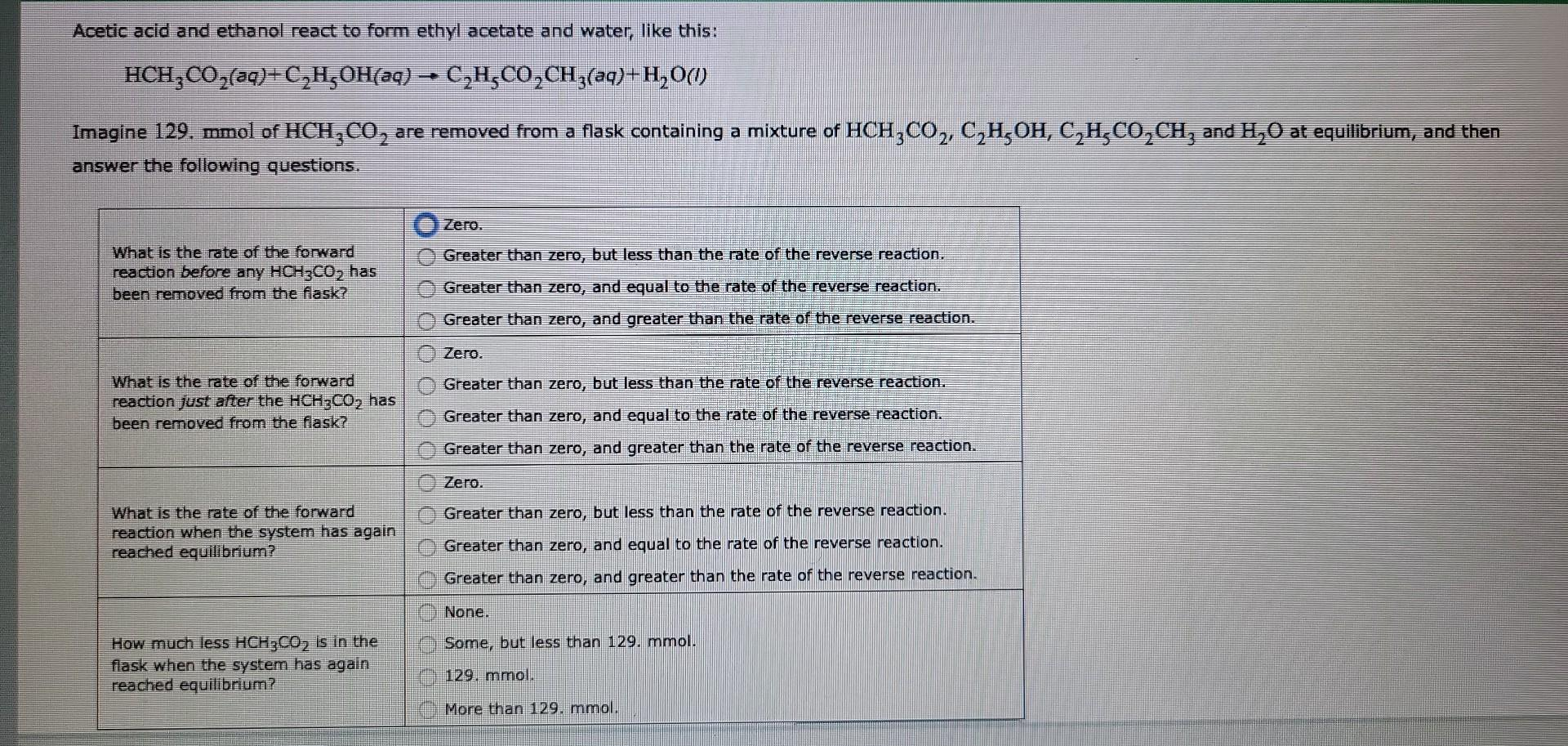Home / Expert Answers / Chemistry / nbsp-acetic-acid-and-ethanol-react-to-form-ethyl-acetate-and-water-like-this-mathrm-hch-pa294

# (Solved):   Acetic acid and ethanol react to form ethyl acetate and water, like this: $\mathrm{HCH}_ ...Acetic acid and ethanol react to form ethyl acetate and water, like this: \[ \mathrm{HCH}_{3} \mathrm{CO}_{2}(a q)+\mathrm{C}_{2} \mathrm{H}_{5} \mathrm{OH}(a q) \rightarrow \mathrm{C}_{2} \mathrm{H}_{5} \mathrm{CO}_{2} \mathrm{CH}_{3}(a q)+\mathrm{H}_{2} \mathrm{O}(i)$ Imagine 129. mmol of $$\mathrm{HCH}_{3} \mathrm{CO}_{2}$$ are removed from a flask containing a mixture of $$\mathrm{HCH}_{3} \mathrm{CO}_{2}, \mathrm{C}_{2} \mathrm{H}_{5} \mathrm{OH}_{2} \mathrm{C}_{2} \mathrm{H}_{5} \mathrm{CO}_{2} \mathrm{CH}_{3}$$ and $$\mathrm{H}_{2} \mathrm{O}_{2}$$ at equilibrium, and then answer the following questions.

We have an Answer from Expert